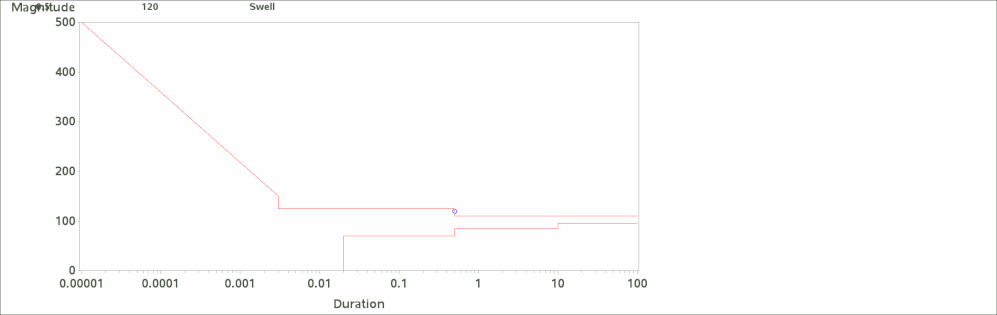## How to create a data table connected to a graph

I have a Query regarding my code for a graph created in SAS.(PFA the html output for the same)

I have created a CBEMA curve graph in Base SAS. This chart generates events (data points) dynamically in a particular time frame.

My program generates an animated gif to show data events occuring dynamically.

Requirement:

My requirement now is that I want a data table above the graph, populated with the latest event(data point) generated on the graph.
I managed to generate the table, but as you can see in the attached html output, the table is getting created over the chart. I want the table to be aligned properly above the graph and the chart below it.

Code functioning:
I have annotated the Cbema curve and the Data table separately in the code and have set both the annotated tables in one table named “anno_all “ and have called it in the proc gchart procedure.

According to my knowledge because of this my table is getting created over the chart.

So I want to know if it’s possible to call both the annotated tables(annocbema and anno_table) separately in the graph?

Is there any other method with which I can achieve my requirement.

Pasting below the macro I created to generate the attached graph:

%macro iterate;
data _null_;
if 0 then set work.cbema nobs=nobs;
call symput ('n',nobs);
run;
%do i=1 %to &n;
set work.cbema (obs=&i);
run;
proc sql print;
create table events as
select Duration, Magnitude, Event from read&i;
quit;
data anno_table; set events;
length text \$10;
function ="frame"; output;
xsys='3'; ysys='5'; hsys='1'; size=4; when='a'; function='label';
y=100-_n_*5;
x=5; position='1'; text=trim(left(Duration)); output;
x=15; position='2'; text=trim(left(Magnitude)); output;
x=25; position='3'; text=trim(left(Event)); output;
run;
data anno_all; set anno_table annocbema;
run;
plot magnitude*duration / annotate = anno_all haxis = axis1 vaxis = axis2 html=tips1;
plot2 magnitude*incident /
haxis = axis1 vaxis = axis2 html=tips1/*noframe*/
autovref cvref=graydd
autohref chref=graydd

des="" name="&name" ;

run;

output;
%end;
%mend;

Any kind of help will be appreciated.

ThanksDiscussion stats
• 0 replies
• 863 views
• 0 likes
• 1 in conversation